Action Reaction

A workshop presented on May 1, 2004 by Paul Doherty

For every action there is an equal and opposite reaction

The most important single idea about action and reaction forces is this:

Action and reaction forces always operate on different objects.

One of the most important discoveries of Isaac Newton, his third law, was that forces always occurred in pairs, one force of the pair was called the action and the other the reaction. Newton's law was that the action and the reaction forces are equal and opposite.

If we want to know how an object moves or distorts its shape we need to find all of the forces on that object. This means we have to define an object, I do this by drawing by drawing a force isolation diagram, in the diagram I mentally isolate the object I want to study and I draw all of the forces on that object. Each and every one of these forces is an action force. Each and every one of these forces has a reaction force that is exerted on another object!

For example: Consider a stone sitting on the ground at rest. (A rather Zen thing to do.)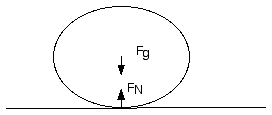A force isolation diagram of a stone sitting at rest.

There are two equal and opposite forces on the stone the force of gravity from the earth Fg and the normal force of the earth's surface on the stone FN, this force is also called the contact force. These forces are equal and opposite but they are not action/reaction pairs. The sum of the forces is zero and so the stone has no acceleration since the net force on the stone is zero. Each of these forces is an action force and each has an equal and opposite reaction force.

Here I show the reaction forces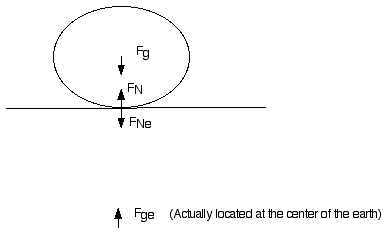This is no longer a proper force isolation diagram since it shows forces on more than one object.

The reaction force to the force of gravity of the earth on the stone, Fg , is the force of gravity of the stone on the earth, Fge. These forces are equal and opposite. The reaction force to the normal force between the surface and the stone, FN , is the contact force of the stone on the earth, FNe.

A Tricky Question

Consider a tug of war between two people, a rope connects them.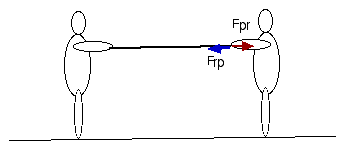A tug of war showing two equal and opposite forces.

Since the force of a person on the rope and the force of the rope on the person are equal and opposite how can anyone ever win the tug of war?

Did you catch the problem?

To find the motion of an object you must consider the forces on that object, this problem states the forces on two different objects the rope and the person.

Not a trick question.

Are the forces mentioned above and action/reaction pair?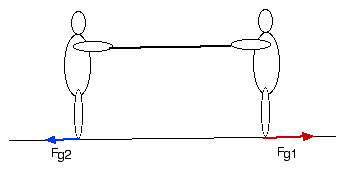A tug of war showing the horizontal forces that actually matter. These forces are not equal and opposite.

In the above drawing I show the horizontal forces that mater in a tug of war.

What is the object that these forces act on?

Answers: Yes the forces are an action/reaction pair. The object is made up of two people and a rope.

Force Scales

Take two scales. Hook them together.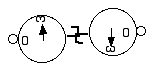Two scales hooked together always read the same forces.

Pull on the scales.

Compare the readings on the scales.

What exactly do scales measure? The scales measure the force applied to the hook.

They do not measure the net force on the scale which is usually zero.

The forces measured by the two scales are an action//reaction pair.

A person pulls on a scale which is attached to a bolt in the wall with a cord, the scale reads 500N.

A person pulls on a scale which is attached by a cord to another scale held by a second person, the scale reads 500N. Compare the forces in these two situations, find the action reaction pairs.

Rocket Propulsion

The most important application of Action/Reaction.

Inflate a balloon. Let it go with. Observe the motion of the balloon through the air of the room.

Predict how the balloon would move in the vacuum of space.

In the early 20'th century Robert Goddard was experimenting with liquid fueled rockets.

He hypothesized that these rockets could be used to travel through the vacuum of space.

The New York Times published an article in 1920 that called his claims ridiculous since there was no air for the rocket exhaust to push against in outer space. As anyone knew, the paper explained with an editorial eye roll, space travel was impossible, since without atmosphere to push against, a rocket could not move so much as an inch. Professor Goddard, it was clear, lacked "the knowledge ladled out daily in high schools."

The Times had missed action/reaction forces.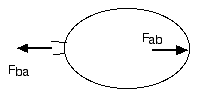A net force on the air accelerates it out of the balloon, A reaction force accelerates the balloon.
This is not a force isolation diagram.

The air inside the balloon is under pressure greater than atmospheric pressure due to the compression by the stretched rubber skin. When the inflator valve is opened the air in the balloon accelerates out under the action of the high pressure inside the balloon and the low pressure outside. The action force is the force of the balloon on the air, the reaction force is the force of the air on the balloon. This reaction force propels the balloon in the opposite direction from the exhaust gasses.

After the Apollo moon landing, the New York Times took a backward glance at its attack on Robert Goddard, published 49 years before. "Further investigation and experimentation," said the paper in 1969, "have confirmed the findings of Isaac Newton in the 17th century, and it is now definitely established that a rocket can function in a vacuum as well as in an atmosphere. The Times regrets the error.

Magnet collisions

Check out the magnet collision activity an the magnetic atmosphere model activity.

Magnetic atmosphere model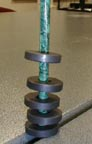A model of the atmosphere using donut magnets on a pencil.

Magnetic atmosphere model: Place two donut magnets on a pencil so that they repel. Then stack 3, 4 and 5. Notice the spacing between the magnets, they are close together at the bottom of the stack and far apart at the top. This is the same as the spacing of molecules in the atmosphere of a planet.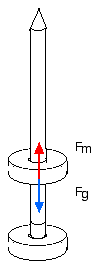A force isolation diagram for a magnet suspended above another magnet on a pencil.
.Fg is the force of gravity on the top magnet, its weight, while Fm is the force from the bottom magnet.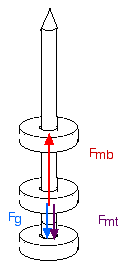A stack of three magnets. Fmt is the force of the top magnet on the middle magnet, Fg is the force of gravity on the middle magnet., and Fmb is the force of the bottom magnet on the middle one. The force Fmb must be larger so the magnets must be closer.

In this drawing the force of the top magnet on the middle one, Fmt, is the reaction force to the force of the middle one on the top one. The force on the top magnet by the middle magnet must support it against gravity. So the bottom magnet must exert a force on the middle magnet that is large enough to support the weight of two magnets.

Now you can figure out all the forces in the stack of 5 magnets in the photo.

Magnet Collisions

The forces on the two magnets as the top magnet falls under gravity toward the bottom one.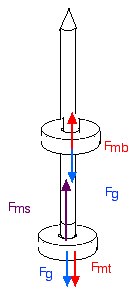The forces on the two magnets in the silent collision activity in which the top magnet is dropped onto the first.
Fg is the force of gravity, Fms is the force of steel eraser on the magnet, Fmb is the force of the bottom magnet on the top, and Fmt is the force of the top on the bottom.

There is an action reaction pair of forces shown in this drawing. Which forces are they?

What is the reaction force to Fms?

Answers: The action reaction pair of forces is Fmt and Fmb .

The reaction force to Fms is the force of the magnet on the steel eraser.

Film can rocket

A rocket can be made with a film can.

The best film cans to use are the white ones with thick tops, the worst ones are the black film cans which often leak gas.

To Do and Notice

Put water into the film can until it is 1/4 full.

Put baking powder into the depression that is inside the lid.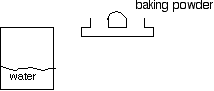Fill the lid with baking powder and then put water into the film can.

Put the lid on the film can, place it on the ground and step back. After ten seconds there will be a "pop" and the film can lid will be launched into the air.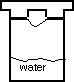Put the lid on the can and step back.

What's Going On?

Baking powder contains a dry tartaric acid powder in addition to bicarbonate of soda. Whe wet by water the tartaric acid dissolves and reacts with the bicarbonate releasing carbon dioxide gas. The pressure builds inside the can. When the pressure is great enough the lid of the film can is blasted into the air.

Going Further

What happens if you put the lid on the floor and the film can upright?

What happens when you put the film can on its side?

Try various amounts of water, which yields the greatest height?

You can also use vinegar and baking soda, however the reaction proceeds so rapidly that you may not have time to escape before the lid blasts off.

Try warm water and cold water.

A small car collides with a large truck head-on.

Discuss the force that the truck exerts on the car and the force that the car exerts on the truck.

Solution: these forces are an action-reaction pair.